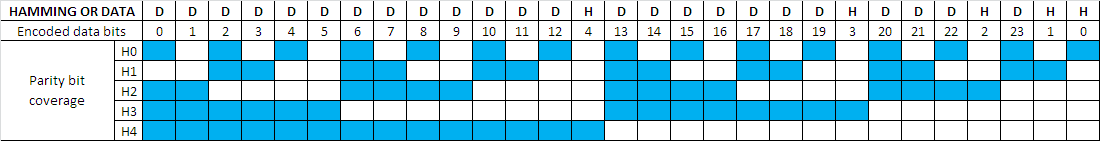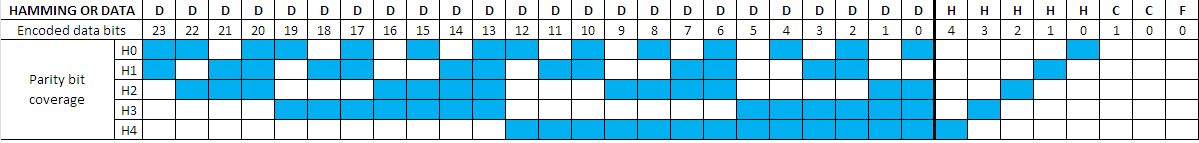SBAA106A June   2020  – August 2021

2. 1Introduction
3. 2Simple Checksum
4. 3CRC
1. 3.1 CRC Generic Computations
1. 3.1.1 Using XOR Bitwise Computation
2. 3.1.2 Using Lookup Tables
3. 3.1.3 CRC Computation Differences Between the ADS122U04 and ADS122C04
5. 4Hamming Code
1. 4.1 Hamming Code Computation
1. 4.1.1 Hamming Code Computation Example
2. 4.1.2 Validation of Transmitted Data
6. 5Summary
7. 6References
8. 7Revision History

# 4 Hamming Code

The discussion of Hamming Code (HC) is limited to how the code is used for data integrity checks for ADCs. HC is used to correct one bit of error for a transmitted data block. Hamming codes work well for verifying small data packets. HC cannot detect all multiple bit errors, and multiple bit errors cannot be corrected. The implementation of the Hamming byte used for the ADS131A0x devices includes the HC as well as checksum bits for determining multiple bit error.

For the ADS131A0x devices the HC is made up of five bits (H0-H4) and the bits are interleaved with the data as shown in Figure 4-1. Parity is used to determine the value of each bit in the HC relative to specific bits mapped to the data. Conceptually, the HC bits are interleaved within the data bits. The result is multiple Hamming bits are covering each data bit. This is an improvement upon early data communication when only a single parity was used for data validation. The HC now uses multiple bits to find and correct a single-bit error. Simple parity computation involves counting the number of 1's in a data packet where the parity bit will be either one or zero depending on whether the parity is considered to be odd or even. Odd parity correlates to an odd count, and even parity to an even count. The Hamming bit is set to make the count even parity for the ADS131A0x devices.

The data word for the ADS131A0x family of devices can be either 16-bits or 24-bits. The Hamming byte consists of five Hamming bits with H4 as the most significant bit. Following the Hamming bits are two checksum bits. The least significant bit of the Hamming byte is a constant equal to zero. The total transfer of the data word and the Hamming byte can be either 24-bits or 32-bits. For this discussion we will consider the 32-bit word length consisting of the 24-bits of data along with the Hamming byte.

The five Hamming bits become a part of the data word and are compared to the parity representation of each bit so that the combination of the Hamming parity covers the data bits at least twice so that if a bit is incorrect it can be reconstructed from the remaining Hamming parity bits. For checking if multiple bits have error, then the 2-bit checksum data is used to verify if the result shows a single bit error, or multiple bit error.Figure 4-1 ADS131A0x Hamming Codes as Computed

The table data in Figure 4-1 shows the parity bit coverage for each Hamming bit with the Hamming bits interleaved within the data. Each row of the table indicates which data bits are included in each Hamming bit. Note that the table is showing the bits with the least significant bit at the left-hand side of the table and the most significant at the right-hand side. The bits that are checked are indicated in the columns, and the rows are representing the Hamming parity bit. Information from the table can be used to determine a Hamming mask for each Hamming bit. The Hamming mask is used to validate the Hamming bit associated for the mask. The mask only includes the data bits used for a particular Hamming bit. When converting the table to the Hamming mask used in computation, the 32-bit values are read from left to right and the data would exclude the Hamming bits in the mask.

Transmission of the data to and from the ADS131A0x devices is most significant bit (msb) first through the least significant bit (lsb) of the data. The Hamming bits will follow in the byte after the data are transmitted. The Hamming is not interleaved within the output as shown in the Figure 4-1. Instead the Hamming is appended to the transmission of the device data as a separate byte. The Hamming bit values will still follow the same pattern and computation but the placing of the Hamming byte following the data is by design. Placing the Hamming byte as the least significant byte (LSB) transmitted allows the user to easily ignore the byte if desired or not transmitted at all if disabled. This allows the device data to appear the same independent of having the Hamming enabled. The Hamming byte also contains the checksum of the data as a 2-bit value (C1 and C0) representing the number of bits set to "1". The least-significant bit (F0) is a fixed constant with a value of "0". The appearance of the transmitted data and Hamming byte will follow the format shown in Figure 4-2.Figure 4-2 ADS131A0x Data Hamming Code as Transmitted

When computing the Hamming bit parity, each Hamming bit will follow the pattern shown in Table 4-1 where each bit can be determined by masking out the bits not computed. Using this method greatly simplifies the computation for verifying the data transmission.

Table 4-1 Hamming Bit Mask
HAMMING BIT MASK VALUE (32-bit)
H000DAB555h
H100B66CCCh
H20071E3C3h
H3000FE03Fh
H400001FFFh

The mask is applied to the data multiple times while counting the number of "1" bits and comparing to the appropriate Hamming parity bit. The masking of the bits is allowed as no Hamming bit includes any other Hamming bit in the parity computation. Also, every data bit is checked by more than one Hamming bit. A Hamming bit error could occur, but will be indicated if the data bits are verified within the other Hamming bit checks. If a data bit is in error, there will be more than one Hamming bit indicating the error in the parity check. When the parity computation is compared to the Hamming bits, the difference will point directly to the data bit in error.

To demonstrate the computation, a 24-bit data example is shown using the value of AC9538h. The results are shown in Table 4-2 for each of the Hamming bits. The process of computation is as follows:

1. H0 computation: Apply mask of H0 to the data (AC9538h & 00DAB55h = 889510h)
2. Find the parity for the result in step 1 (889510h has 7 ones, so parity is odd and H0 becomes 1 to make the total parity even)
3. Repeat steps 1 and 2 for H1 through H4 Hamming bits

Table 4-2 Hamming Bit Computation Example
HAMMING BITDATA (HEX)MASK (HEX)RESULT (HEX)RESULT (BINARY)BIT COUNTHAMMING VALUE
H0AC 95 38DA B5 5588 95 101000 1000 1001 0101 0001 00007 (odd)1
H1AC 95 38B6 6C CCA4 04 081010 0100 0000 0100 0000 10005 (odd)1
H2AC 95 3871 E3 C320 81 000010 0000 1000 0001 0000 00003 (odd)1
H3AC 95 380F E0 3F0C80 380000 1100 1000 0000 0011 10006 (even)0
H4AC 95 3800 1F FF00 15 380000 0000 0001 0101 0011 10006 (even)0

The computation of the checksum bits is based on the number of "1" values in the binary representation of the data. Continuing to use the hex data of AC 95 38h, the hex value is converted to binary and the number of "1"s counted that represent the value. The binary conversion results in "1010 1100 1001 0101 0011 1000b" and the number of "1"s represented is decimal 11. The value of 11 in binary is "1011b." The checksum is computed by adding all 24 data bits with the modulo of 4. To simplify the computation and avoiding the modulo 4 math only the two least significant bits are used as the checksum bits. For this example the checksum is simply "11b".

The entire Hamming/checksum byte would appear in the format shown in Figure 4-2, which is H4, H3, H2, H1, H0, C1, C0, 0. For the example using 0xAC9538, the bit binary representation of the Hamming/checksum byte becomes "0011 1110b".